## CGAL::Triangulation_2<Traits,Tds>

### Definition

The class Triangulation_2<Traits,Tds> is the basic class designed to handle triangulations of set of points  A in the plane.

Such a triangulation has vertices at the points of  A and its domain covers the convex hull of  A. It can be viewed as a planar partition of the plane whose bounded faces are triangular and cover the convex hull of  A. The single unbounded face of this partition is the complementary of the convex hull of  A.

In many applications, it is convenient to deal only with triangular faces. Therefore, we add to the triangulation a fictitious vertex, called the infinite vertex and we make each convex hull edge incident to an infinite face having as third vertex the infinite vertex. In that way, each edge is incident to exactly two faces and special cases at the boundary of the convex hull are simpler to deal with.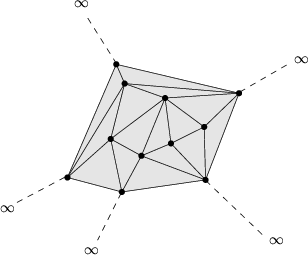Figure 25.9:  The infinite vertex.

The class Triangulation_2<Traits,Tds> implements this point of view and therefore considers the triangulation of the set of points as a set of triangular, finite and infinite faces. Although it is convenient to draw a triangulation as in figure 25.9, note that the infinite vertex has no significant coordinates and that no geometric predicate can be applied on it or on an infinite face.

A triangulation is a collection of vertices and faces that are linked together through incidence and adjacency relations. Each face give access to its three incident vertices and to its three adjacent faces. Each vertex give access to one of its incident faces.

The three vertices of a face are indexed with 0, 1 and 2 in counterclockwise order. The neighbor of a face are also indexed with 0,1,2 in such a way that the neighbor indexed by i is opposite to the vertex with the same index.

The triangulation class offer two functions int cw(int i) and int ccw(int i) which given the index of a vertex in a face compute the index of the next vertex of the same face in clockwise or counterclockwise order. Thus, for example the neighbor neighbor(cw(i)) is the neighbor of f which is next to neighbor(i) turning clockwise around f. The face neighbor(cw(i)) is also the first face encountered after f when turning clockwise around vertex i of f (see Figure 25.10).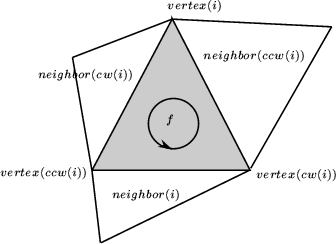Figure 25.10:  Vertices and neighbors.

#include <CGAL/Triangulation_2.h>

### Parameters

The class Triangulation_2<Traits,Tds> has two template parameters. The first one Traits is the geometric traits, it is to be instantiated by a model of the concept TriangulationTraits_2.

The second parameter is the triangulation data structure, it has to be instantiated by a model of the concept TriangulationDataStructure_2. By default, the triangulation data structure is instantiated by CGAL::Triangulation_data_structure_2 < CGAL::Triangulation_vertex_base_2<Gt>, CGAL::Triangulation_face_base_2<Gt> > >.

### Inherits From

Triangulation_cw_ccw_2 This class provides the functions cw(i) and ccw(i).

### Types

 typedef Traits Geom_traits; the traits class. typedef Tds Triangulation_data_structure; the triangulation data structure type. typedef Traits::Point_2 Point; the point type typedef Traits::Segment_2 Segment; the segment type typedef Traits::Triangle_2 Triangle; the triangle type typedef Tds::Vertex Vertex; the vertex type. typedef Tds::Face Face; the face type. typedef Tds::Edge Edge; the edge type. typedef Tds::size_type size_type; Size type (an unsigned integral type) typedef Tds::difference_type difference_type; Difference type (a signed integral type)

The vertices and faces of the triangulations are accessed through handles, iterators and circulators. The handles are models of the concept Handle which basically offers the two dereference operators * and ->. The iterators and circulators are all bidirectional and non mutable. The circulators and iterators are convertible to handles with the same value type, so that whenever a handle appear in the parameter list of a function, an appropriate iterator or circulator can be passed as well.

The edges of the triangulation can also be visited through iterators and circulators, the edge circulators and iterators are also bidirectional and non mutable.

In the following, we called infinite any face or edge incident to the infinite vertex and the infinite vertex itself. Any other feature (face, edge or vertex) of the triangulation is said to be finite. Some iterators (the All iterators ) allows to visit finite or infinite feature while others (the Finite iterators) visit only finite features. Circulators visit infinite features as well as finite ones.

 typedef Tds::Vertex_handle Vertex_handle; handle to a vertex typedef Tds::Face_handle Face_handle; handle to a face typedef Tds::Face_iterator All_faces_iterator; iterator over all faces. typedef Tds::Edge_iterator All_edges_iterator; iterator over all edges typedef Tds::Vertex_iterator All_vertices_iterator; iterator over all vertices

 Triangulation_2::Finite_faces_iterator iterator over finite faces. Triangulation_2::Finite_edges_iterator iterator over finite edges. Triangulation_2::Finite_vertices_iterator iterator over finite vertices. Triangulation_2::Point_iterator iterator over the points corresponding the finite vertices of the triangulation. Triangulation_2::Line_face_circulator circulator over all faces intersected by a line. Triangulation_2::Face_circulator circulator over all faces incident to a given vertex. Triangulation_2::Edge_circulator circulator over all edges incident to a given vertex. Triangulation_2::Vertex_circulator circulator over all vertices incident to a given vertex.

The triangulation class also defines the following enum type to specify which case occurs when locating a point in the triangulation.

 enum Locate_type { VERTEX=0, EDGE, FACE, OUTSIDE_CONVEX_HULL, OUTSIDE_AFFINE_HULL}; The locate type is OUTSIDE_CONVEX_HULL when the point is outside the convex hull but in the affine hull of the current triangulation. The locate type is OUTSIDE_AFFINE_HULL when the point is outside the affine hull of the current triangulation.

### Creation

 Triangulation_2 t; default constructor. Triangulation_2 t ( Traits gt = Traits()); Introduces an empty triangulation t. Triangulation_2 t ( Triangulation_2 tr); Copy constructor. All the vertices and faces are duplicated. After the copy, t and tr refer to different triangulations : if tr is modified, t is not.

 Triangulation_2 t = tr Assignment. All the vertices and faces are duplicated. After the assignment, t and tr refer to different triangulations : if tr is modified, t is not. void t.swap ( Triangulation_2& tr) The triangulations tr and t are swapped. t.swap(tr) should be preferred to t = tr or to t(tr) if tr is deleted after that. void t.clear () Deletes all faces and finite vertices resulting in an empty triangulation.

### Access Functions

 Geom_traits t.geom_traits () Returns a const reference to the triangulation traits object. TriangulationDataStructure_2 t.tds () Returns a const reference to the triangulation data structure.advanced### Non const access

The responsibility of keeping a valid triangulation belongs to the user when using advanced operations allowing a direct manipulation of the tds.

 TriangulationDataStructure_2 & t.tds () Returns a reference to the triangulation data structure.

This method is mainly a help for users implementing their own triangulation algorithms.advancedint t.dimension () Returns the dimension of the convex hull. size_type t.number_of_vertices () Returns the number of finite vertices. size_type t.number_of_faces () Returns the number of finite faces. Face_handle t.infinite_face () a face incident to the infinite_vertex. Vertex_handle t.infinite_vertex () the infinite_vertex. Vertex_handle t.finite_vertex () a vertex distinct from the infinite_vertex.

### Predicates

The class Triangulation_2<Traits,Tds> provides methods to test the finite or infinite character of any feature, and also methods to test the presence in the triangulation of a particular feature (edge or face).

 bool t.is_infinite ( Vertex_handle v) true iff v is the infinite_vertex. bool t.is_infinite ( Face_handle f) true iff face f is infinite. bool t.is_infinite ( Face_handle f, int i) true iff edge (f,i) is infinite. bool t.is_infinite ( Edge e) true iff edge e is infinite. bool t.is_infinite ( Edge_circulator ec) true iff edge *ec is infinite. bool t.is_infinite ( Edge_iterator ei) true iff edge *ei is infinite. bool t.is_edge ( Vertex_handle va, Vertex_handle vb) true if there is an edge having va and vb as vertices. bool t.is_edge ( Vertex_handle va, Vertex_handle vb, Face_handle& fr, int & i) as above. In addition, if true is returned, the edge with vertices va and vb is the edge e=(fr,i) where fr is a handle to the face incident to e and on the right side of e oriented from va to vb. bool t.includes_edge ( Vertex_handle va, Vertex_handle & vb, Face_handle& fr, int & i) true if the line segment from va to vb includes an edge e incident to va. If true, vb becomes the other vertex of e, e is the edge (fr,i) where fr is a handle to the face incident to e and on the right side e oriented from va to vb. bool t.is_face ( Vertex_handle v1, Vertex_handle v2, Vertex_handle v3) true if there is a face having v1, v2 and v3 as vertices. bool t.is_face ( Vertex_handle v1, Vertex_handle v2, Vertex_handle v3, Face_handle &fr) as above. In addition, if true is returned, fr is a handle to the face with v1, v2 and v3 as vertices.

### Queries

The class Triangulation_2<Traits,Tds> provides methods to locate a given point with respect to a triangulation. It also provides methods to locate a point with respect to a given finite face of the triangulation.

Face_handle t.locate ( Point query, Face_handle f = Face_handle())
If the point query lies inside the convex hull of the points, a face that contains the query in its interior or on its boundary is returned.
If the point query lies outside the convex hull of the triangulation but in the affine hull, the returned face is an infinite face which is a proof of the point's location :
- for a two dimensional triangulation, it is a face (, p, q) such that query lies to the left of the oriented line pq (the rest of the triangulation lying to the right of this line).
- for a degenerate one dimensional triangulation it is the (degenerate one dimensional) face (, p, NULL) such that query and the triangulation lie on either side of p.
If the point query lies outside the affine hull, the returned Face_handle is NULL.
The optional Face_handle argument, if provided, is used as a hint of where the locate process has to start its search.

Face_handle t.locate ( Point query, Locate_type& lt, int& li, Face_handle h =Face_handle())
Same as above. Additionally, the parameters lt and li describe where the query point is located. The variable lt is set to the locate type of the query. If lt==VERTEX the variable li is set to the index of the vertex, and if lt==EDGE li is set to the index of the vertex opposite to the edge. Be careful that li has no meaning when the query type is FACE, OUTSIDE_CONVEX_HULL, or OUTSIDE_AFFINE_HULL or when the triangulation is 0-dimensional.

Oriented_side t.oriented_side ( Face_handle f, Point p)
Returns on which side of the oriented boundary of f lies the point p.
 Precondition: f is finite.

Oriented_side t.side_of_oriented_circle ( Face_handle f, Point p)
Returns on which side of the circumcircle of face f lies the point p. The circle is assumed to be counterclockwise oriented, so its positive side correspond to its bounded side. This predicate is available only if the corresponding predicates on points is provided in the geometric traits class.

### Modifiers

The following operations are guaranteed to lead to a valid triangulation when they are applied on a valid triangulation.

void t.flip ( Face_handle f, int i) Exchanges the edge incident to f and f->neighbor(i) with the other diagonal of the quadrilateral formed by f and f->neighbor(i).
 Precondition: The faces f and f->neighbor(i) are finite faces and their union form a convex quadrilateral.

Vertex_handle t.insert ( Point p, Face_handle f = Face_handle())
Inserts point p in the triangulation and returns the corresponding vertex.
If point p coincides with an already existing vertex, this vertex is returned and the triangulation remains unchanged.
If point p is on an edge, the two incident faces are split in two.
If point p is strictly inside a face of the triangulation, the face is split in three.
If point p is strictly outside the convex hull, p is linked to all visible points on the convex hull to form the new triangulation.
At last, if p is outside the affine hull (in case of degenerate 1-dimensional or 0-dimensional triangulations), p is linked all the other vertices to form a triangulation whose dimension is increased by one. The last argument f is an indication to the underlying locate algorithm of where to start.

Vertex_handle t.insert ( Point p, Locate_type lt, Face_handle loc, int li)
Same as above except that the location of the point p to be inserted is assumed to be given by (lt,loc,i) (see the description of the locate method above.)

Vertex_handle t.push_back ( Point p) Equivalent to insert(p).

template < class InputIterator >
int t.insert ( InputIterator first, InputIterator last)
Inserts the points in the range [.first, last.). Returns the number of inserted points.
 Precondition: The value_type of InputIterator is Point.

void t.remove ( Vertex_handle v) Removes the vertex from the triangulation. The created hole is re-triangulated.
 Precondition: Vertex v must be finite.Figure 25.11:  Insertion of a point on an edge.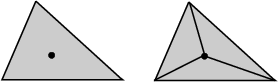Figure 25.12:  Insertion in a face.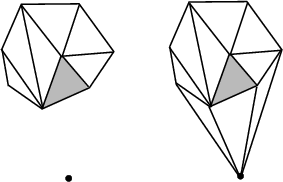Figure 25.13:  Insertion outside the convex hull.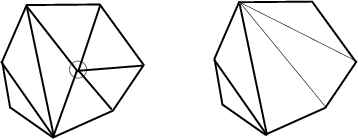Figure 25.14:  RemovaladvancedThe following member functions offer more specialized versions of the insertion or removal operations to be used when one knows to be in the corresponding case.

Vertex_handle t.insert_first ( Point p) Inserts the first finite vertex .

Vertex_handle t.insert_second ( Point p) Inserts the second finite vertex .

Vertex_handle t.insert_in_face ( Point p, Face_handle f)
Inserts vertex v in face f. Face f is modified, two new faces are created.
 Precondition: The point in vertex v lies inside face f.

Vertex_handle t.insert_in_edge ( Point p, Face_handle f, int i)
Inserts vertex v in edge i of f.
 Precondition: The point in vertex v lies on the edge opposite to the vertex i of face f.

Vertex_handle t.insert_outside_convex_hull ( Point p, Face_handle f)
Inserts a point which is outside the convex hull but in the affine hull.
 Precondition: The handle f points to a face which is a proof of the location ofp, see the description of the locate method above.

Vertex_handle t.insert_outside_affine_hull ( Point p)
Inserts a point which is outside the affine hull.

void t.remove_degree_3 ( Vertex_handle v)
Removes a vertex of degree three. Two of the incident faces are destroyed, the third one is modified.
 Precondition: Vertex v is a finite vertex with degree three.

void t.remove_second ( Vertex_handle v)
Removes the before last finite vertex.

void t.remove_first ( Vertex_handle v) Removes the last finite vertex.

The following functions are mainly intended to be used in conjunction with the find_conflicts() member functions of Delaunay and constrained Delaunay triangulations to perform insertions.

 template Vertex_handle t.star_hole ( Point p, EdgeIt edge_begin, EdgeIt edge_end) creates a new vertex v and use it to star the hole whose boundary is described by the sequence of edges [edge_begin, edge_end[. Returns a handle to the new vertex. template Vertex_handle t.star_hole ( Point p, EdgeIt edge_begin, EdgeIt edge_end, FaceIt face_begin, FaceIt face_end) same as above, except that the algorithm first recycles faces in the sequence [face_begin, face_end[ and create new ones only when the sequence is exhausted.advanced### Traversal of the Triangulation

A triangulation can be seen as a container of faces and vertices. Therefore the triangulation provides several iterators and circulators that allow to traverse it (completely or partially).

### Face, Edge and Vertex Iterators

The following iterators allow respectively to visit finite faces, finite edges and finite vertices of the triangulation. These iterators are non mutable, bidirectional and their value types are respectively Face, Edge and Vertex. They are all invalidated by any change in the triangulation.

 Finite_vertices_iterator t.finite_vertices_begin () Starts at an arbitrary finite vertex Finite_vertices_iterator t.finite_vertices_end () Past-the-end iterator Finite_edges_iterator t.finite_edges_begin () Starts at an arbitrary finite edge Finite_edges_iterator t.finite_edges_end () Past-the-end iterator Finite_faces_iterator t.finite_faces_begin () Starts at an arbitrary finite face Finite_faces_iterator t.finite_faces_end () Past-the-end iterator Point_iterator t.points_begin () Point_iterator t.points_end () Past-the-end iterator

The following iterators allow respectively to visit all (finite or infinite) faces, edges and vertices of the triangulation. These iterators are non mutable, bidirectional and their value types are respectively Face, Edge and Vertex. They are all invalidated by any change in the triangulation.

 All_vertices_iterator t.all_vertices_begin () Starts at an arbitrary vertex All_vertices_iterator t.all_vertices_end () Past-the-end iterator All_edges_iterator t.all_edges_begin () Starts at an arbitrary edge All_edges_iterator t.all_edges_end () Past-the-end iterator All_faces_iterator t.all_faces_begin () Starts at an arbitrary face All_faces_iterator t.all_faces_end () Past-the-end iterator

### Line Face Circulator

The triangulation defines a circulator that allows to visit all faces that are intersected by a line. A face f is considered has being intersected by the oriented line l if either:

• f is a finite face whose interior intersects l, or
• f is a finite face with an edge collinear with l and lies to the left of l, or
• f is an infinite face incident to a convex hull edge whose interior is intersected by l, or
• f is an infinite face incident to a convex hull vertex lying on l and the finite edge of f lies to the left of l.
The circulator has a singular value if the line l intersect no finite face of the triangulation. This circulator is non-mutable and bidirectional. Its value type is Face.

Line_face_circulator t.line_walk ( Point p, Point q, Face_handle f = Face_handle())
This function returns a circulator that allows to visit the faces intersected by the line pq. If there is no such face the circulator has a singular value.
The starting point of the circulator is the face f, or the first finite face traversed by l , if f is omitted.
The circulator wraps around the infinite_vertex : after the last traversed finite face, it steps through the infinite face adjacent to this face then through the infinite face adjacent to the first traversed finite face then through the first finite traversed face again.
 Precondition: Points p and q must be different points.
 Precondition: If f != NULL, it must point to a finite face and the point p must be inside or on the boundary of f.

Figure 25.15 illustrates which finite faces are enumerated. Lines l1 and l2 have no face to their left. Lines l3 and l4 have faces to their left. Note that the finite faces that are only vertex incident to lines l3 and l4 are not enumerated.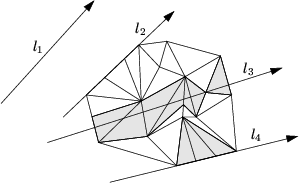Figure 25.15:  The line face circulator.

A line face circulator is invalidated if the face the circulator refers to is changed.

### Face, Edge and Vertex Circulators

The triangulation also provides circulators that allows to visit respectively all faces or edges incident to a given vertex or all vertices adjacent to a given vertex. These circulators are non-mutable and bidirectional. The operator++ moves the circulator counterclockwise around the vertex while the operator-- moves clockwise. A face circulator is invalidated by any modification of the face pointed to. An edge or a vertex circulator are invalidated by any modification of one of the two faces incident to the edge pointed to.

Face_circulator t.incident_faces ( Vertex_handle v)
Starts at an arbitrary face incident to v.
Face_circulator t.incident_faces ( Vertex_handle v, Face_handle f)
Starts at face f.
 Precondition: Face f is incident to vertex v.
Edge_circulator t.incident_edges ( Vertex_handle v)
Starts at an arbitrary edge incident to v.
Edge_circulator t.incident_edges ( Vertex_handle v, Face_handle f)
Starts at the first edge of f incident to v, in counterclockwise order around v.
 Precondition: Face f is incident to vertex v.
Vertex_circulator t.incident_vertices ( Vertex_handle v)
Starts at an arbitrary vertex incident to v.
Vertex_circulator t.incident_vertices ( Vertex_handle v, Face_handle f)
Starts at the first vertex of f adjacent to v in counterclockwise order around v.
 Precondition: Face f is incident to vertex v.

### Traversal of the Convex Hull

Applied on the infinite_vertex the above functions allow to visit the vertices on the convex hull and the infinite edges and faces. Note that a counterclockwise traversal of the vertices adjacent to the infinite_vertex is a clockwise traversal of the convex hull.

 Face_circulator t.incident_faces ( t.infinite_vertex()) Face_circulator t.incident_faces ( t.infinite_vertex(), Face_handle f) Edge_circulator t.incident_edges ( t.infinite_vertex()) Edge_circulator t.incident_edges ( t.infinite_vertex(), Face_handle f) Vertex_circulator t.incident_vertices ( t.infinite_vertex() v) Vertex_circulator t.incident_vertices ( t.infinite_vertex(), Face_handle f)

Vertex_handle t.mirror_vertex ( Face_handle f, int i)
returns the vertex of the ith neighbor of f that is opposite to f.
 Precondition: 0i2.

int t.mirror_index ( Face_handle f, int i)
returns the index of f in its ith neighbor.
 Precondition: 0i2.

### Miscellaneous

int t.ccw ( int i) Returns i+1 modulo 3.
 Precondition: 0i2.
int t.cw ( int i) Returns i+2 modulo 3.
 Precondition: 0i2.
Triangle t.triangle ( Face_handle f) Returns the triangle formed by the three vertices of f.
 Precondition: The face is finite.
Segment t.segment ( Face_handle f, int i) Returns the line segment formed by the vertices ccw(i) and cw(i) of face f.
 Precondition: 0i2. The vertices ccw(i) and cw(i) of f are finite.
Segment t.segment ( Edge e) Returns the line segment corresponding to edge e.
 Precondition: e is a finite edge
Segment t.segment ( Edge_circulator ec) Returns the line segment corresponding to edge *ec.
 Precondition: *ec is a finite edge.
Segment t.segment ( Edge_iterator ei) Returns the line segment corresponding to edge *ei.
 Precondition: *ei is a finite edge.
Point t.circumcenter ( Face_handle f) Compute the circumcenter of the face pointed to by f. This function is available only if the corresponding function is provided in the geometric traits.advanced### Setting

 void t.set_infinite_vertex ( Vertex_handle v)

### Checking

The responsibility of keeping a valid triangulation belongs to the users if advanced operations are used. Obviously the advanced user, who implements higher levels operations may have to make a triangulation invalid at some times. The following method is provided to help the debugging.

 bool t.is_valid ( bool verbose = false, int level = 0) Checks the combinatorial validity of the triangulation and also the validity of its geometric embedding. This method is mainly a debugging help for the users of advanced features.advanced### I/O

The I/O operators are defined for iostream. The format for the iostream is an internal format.

ostream& ostream& os << T Inserts the triangulation t into the stream os.
 Precondition: The insert operator must be defined for Point.

istream& istream& is >> T Reads a triangulation from stream is and assigns it to t.
 Precondition: The extract operator must be defined for Point.

The information output in the iostream is:
- the dimension, the number of vertices (including the infinite one), and the number of faces (including infinite ones).
- for each vertex (except the infinite vertex), the non combinatorial information stored in that vertex (point, etc.).
- for each faces, the indices of its vertices and the non combinatorial information (if any) in this face. - for each face again the indices of the neighboring faces.
The index of an item (vertex of face) is the rank of this item in the output order. When dimension < 2, the same information is output for faces of maximal dimension instead of faces.

CGAL also provides stream operators << to draw triangulations on CGAL::Window_stream, the LEDA based graphic package, and on CGAL::Qt_widget, the Qt based graphic package. These operators requires respectively the include statements :
#include CGAL/IO/Window_stream.h
#include CGAL/IO/Qt_widget_Triangulation_2.h
See the Window_stream and Qt_widget classes.

### Implementation

Locate is implemented by a line walk from a vertex of the face given as optional parameter (or from a finite vertex of infinite_face() if no optional parameter is given). It takes time O(n) in the worst case, but only O(sqrt(n)) on average if the vertices are distributed uniformly at random.

Insertion of a point is done by locating a face that contains the point, and then splitting this face. If the point falls outside the convex hull, the triangulation is restored by flips. Apart from the location, insertion takes a time time O(1). This bound is only an amortized bound for points located outside the convex hull.

Removal of a vertex is done by removing all adjacent triangles, and re-triangulating the hole. Removal takes time O(d^2) in the worst case, if d is the degree of the removed vertex, which is O(1) for a random vertex.

The face, edge, and vertex iterators on finite features are derived from their counterparts visiting all (finite and infinite) features which are themselves derived from the corresponding iterators of the triangulation data structure.